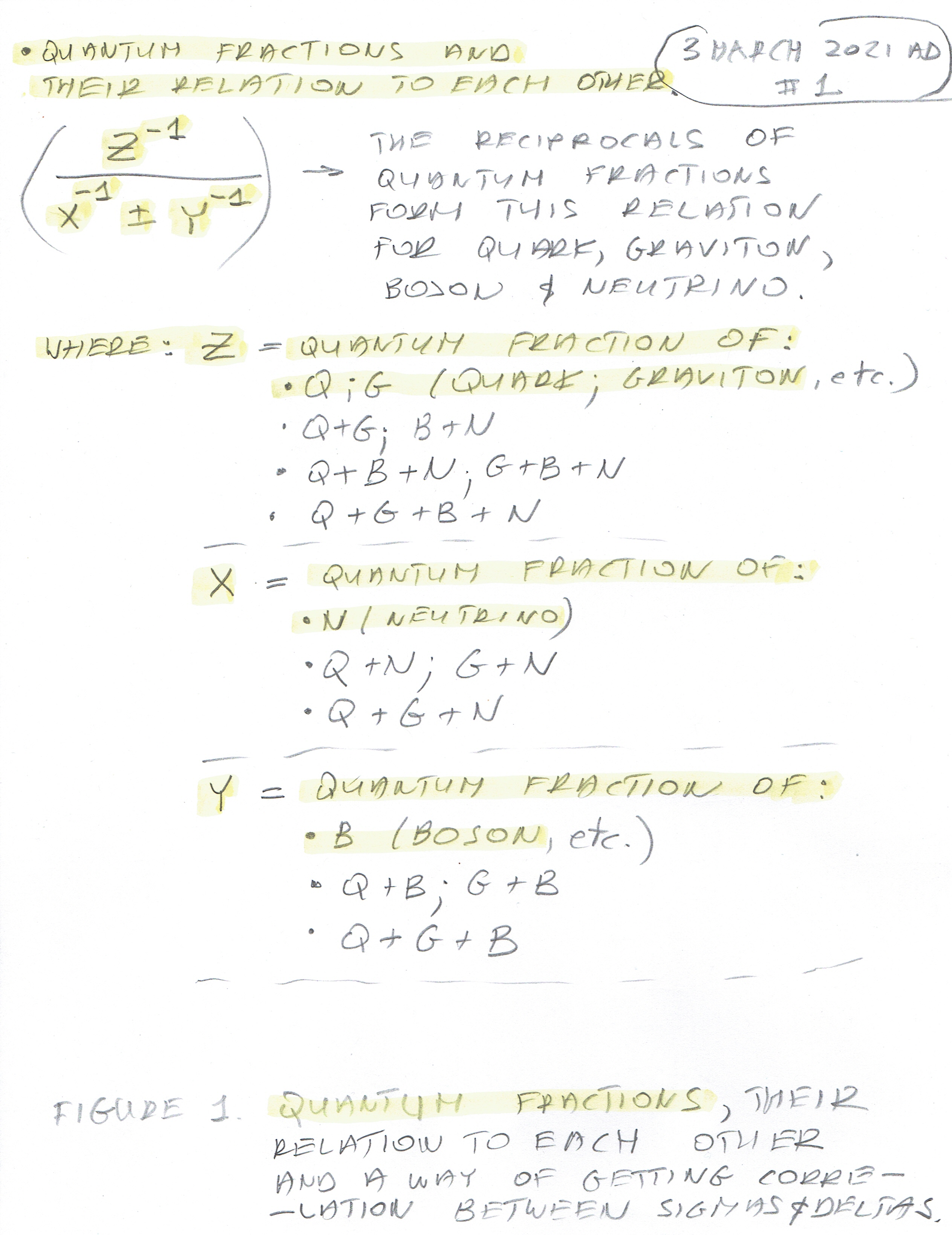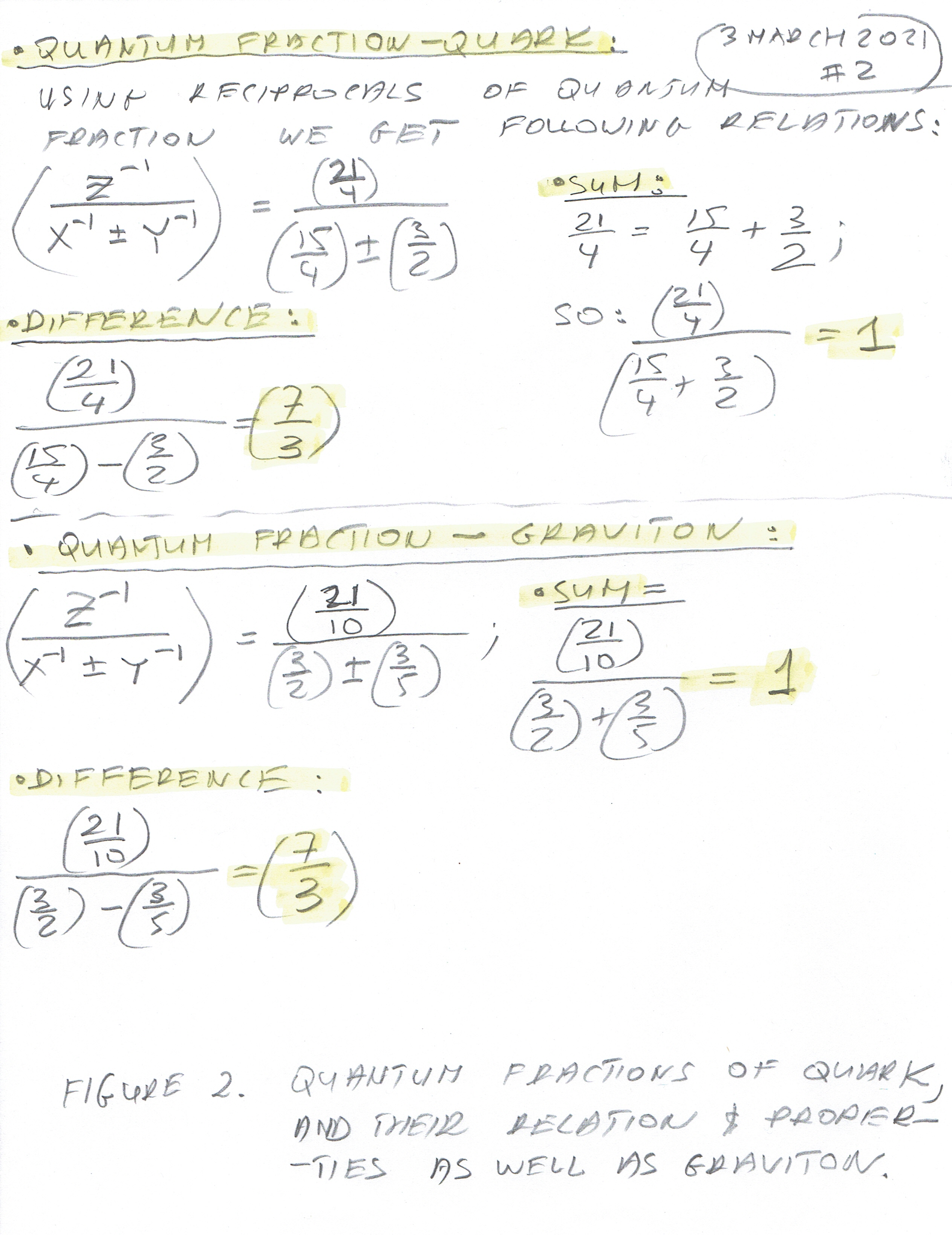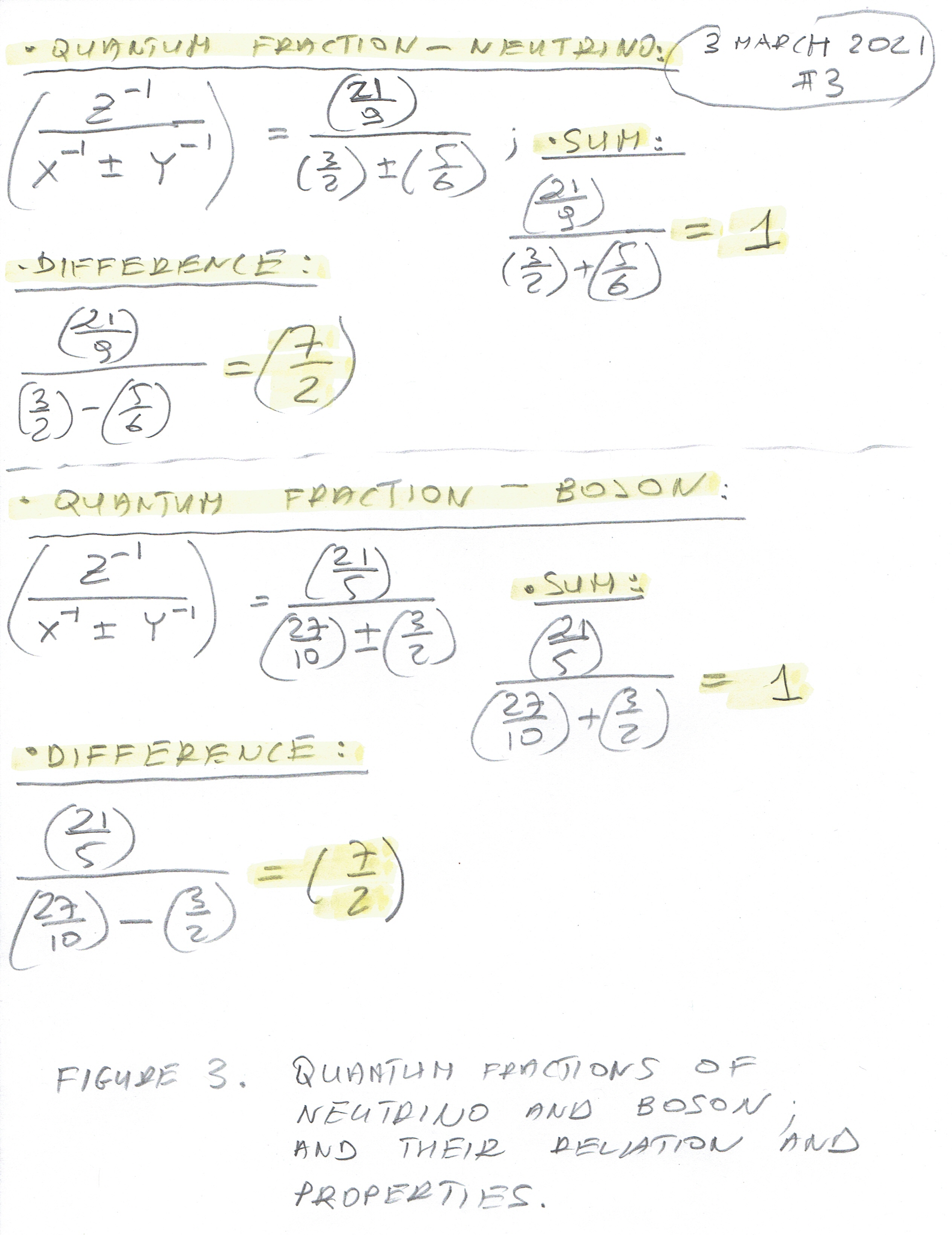4 March 2021 AD

St Casimir of Poland (1484); St Lucius I (254)

This article deals with "Quantum Fractions". Those are numbers that connect Theta Angles (Mixing (Oscillation) Angles) and Delta Angles (CP Violating Phase Angles). These Angles are the sums of all three Mixing (Oscillation) Theta Angles from before and after the Transform into the Positive Real Part of The complex plane - I call these angles "Sigma" since they are the sums.

They are derived from known quantities i.e. Quark and Neutrino Mixing (Oscillation) Angles and CP Violating Phase Angles. Then they are generalized for Boson and Graviton and later for other elements such as Space, Time, Membrane Internal and Membrane External. Quantum Fractions are very simple concepts. There are three of them - for Quark and Graviton and combinations of them, for Neutrino and combinations and for Boson and combinations. I call them: "Z" - Quark and Graviton; "X" - Neutrino and "Y" - Boson. They have all sort of interesting properties. The important property for calculating the relationship between the angles is the quotient of reciprocals: ( Z-1 / ( X-1  + or - Y-1 )).

There are combinations of the sums of the Theta Angles (SIgma) - the can form a single entity, a pair, a triplet and a quadruplet where all four Thetas (Sigmas) are added together. The quadruplet will be used to calculate the CP Violating Phase Angles, (the sum of all Sigmas of Quark, Graviton, Neutrino and Boson adds up to about 540 degrees - i.e. the sum of the internal angles of a pentagon). I think this "average" gives the best approximation of CP Violating Phase Angle Delta, however, any other combination may be used and will give results only slightly different.

Here is Figure 1 - Quantum Fractions and their relations to each other:You can see the general formula Z/(X+Y), and the combinations of all four elements - Quark, Graviton, Neutrino and Boson. The last entry under "Z" consisting of all four elements will be used for calculating or confirming CP Violating Phase Angles (Deltas) of Quark, Graviton, Neutrino and Boson.

The second Figure contains basic relations and concrete values of Quantum Fractions for Quark and Graviton. Notice that the ( Z-1 / ( X-1 - Y-1 ) = ( 7/3 ) for both Quark and Graviton.

Figure 2 - Quantum Fractions pf Quark and Graviton.Figure 3 is similar to Figure 2 - it shows the concrete values of Quantum Fractions for neutrino and Boson. Notice that ( Z-1 / ( X-1 - Y-1 ) = ( 7/2 ) for both of the Neutrino and Boson.

Figure 3 - Quantum Fractions of Neutrino and Boson and their relation and properties.Here are the links to the previous articles on how to get the Quantum Fractions, if you want to read:

65. "Quantum Fraction" of the CP Violation Phase Angle of Neutrino

70. Exact value of the CP Violation Phase Angle of Boson.

80. The Exact value of the CP Violation Phase Angle of Graviton.

Comments powered by CComment